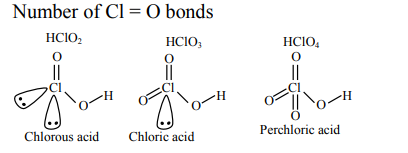# Number of Cl = O bonds in chlorous acid,`
Question:

Number of $\mathrm{Cl}=\mathrm{O}$ bonds in chlorous acid, chloric acid and perchloric acid respectively are :

1. 3,1 and 1

2. 4,1 and 0

3. 1,1 and 3

4. 1,2 and 3

Correct Option: , 3

Solution: